Скачать презентацию DEMAND ANALYSIS AND FORECASTING — Prof V Chandra

9d33b86437275458f84303042301507e.ppt

• Количество слайдов: 15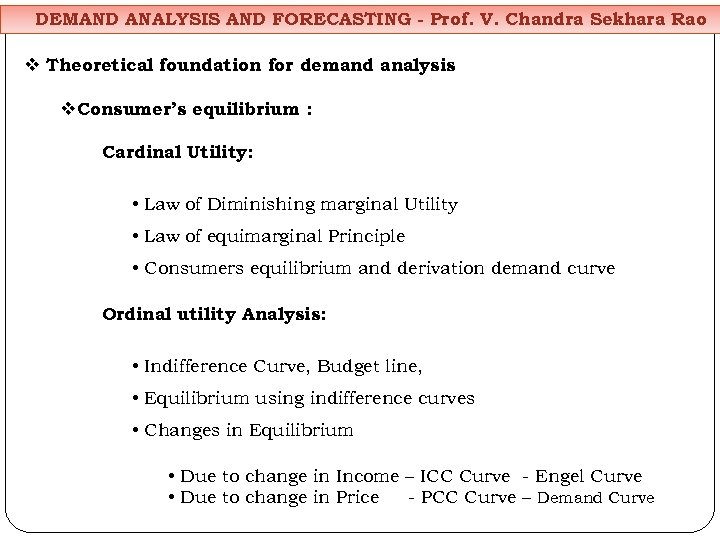DEMAND ANALYSIS AND FORECASTING - Prof. V. Chandra Sekhara Rao v Theoretical foundation for demand analysis v. Consumer’s equilibrium : Cardinal Utility: • Law of Diminishing marginal Utility • Law of equimarginal Principle • Consumers equilibrium and derivation demand curve Ordinal utility Analysis: • Indifference Curve, Budget line, • Equilibrium using indifference curves • Changes in Equilibrium • Due to change in Income – ICC Curve - Engel Curve • Due to change in Price - PCC Curve – Demand Curve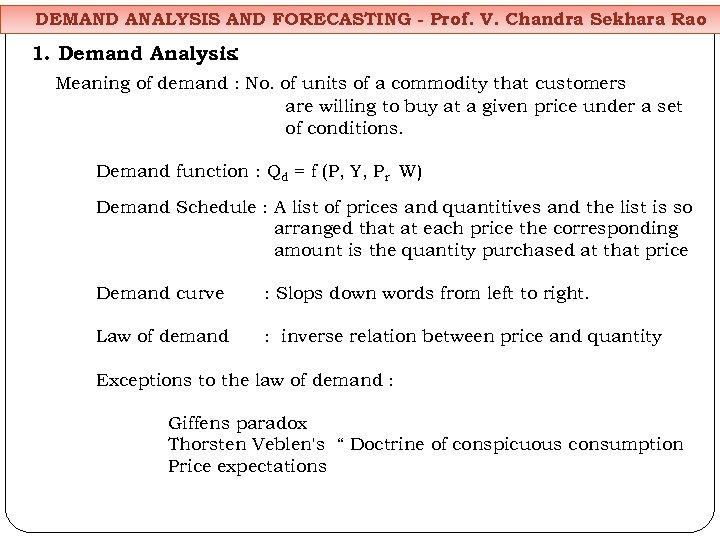DEMAND ANALYSIS AND FORECASTING - Prof. V. Chandra Sekhara Rao 1. Demand Analysis: Meaning of demand : No. of units of a commodity that customers are willing to buy at a given price under a set of conditions. Demand function : Qd = f (P, Y, Pr W) Demand Schedule : A list of prices and quantitives and the list is so arranged that at each price the corresponding amount is the quantity purchased at that price Demand curve : Slops down words from left to right. Law of demand : inverse relation between price and quantity Exceptions to the law of demand : Giffens paradox Thorsten Veblen's “ Doctrine of conspicuous consumption Price expectations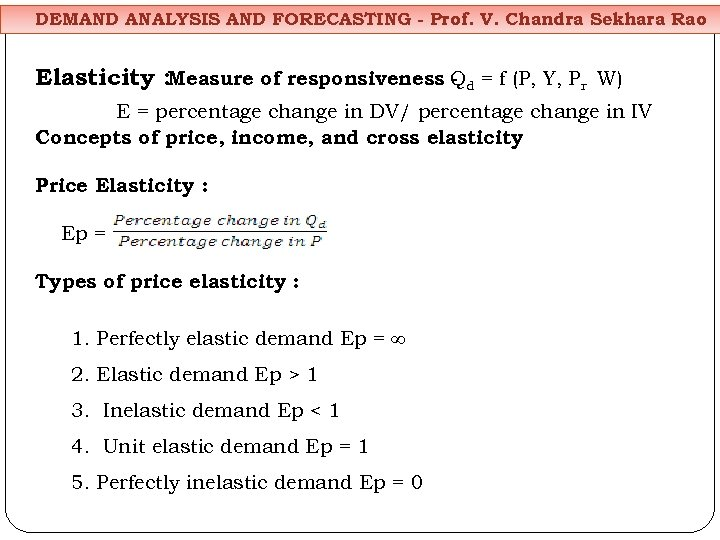DEMAND ANALYSIS AND FORECASTING - Prof. V. Chandra Sekhara Rao Elasticity : Measure of responsiveness Qd = f (P, Y, Pr W) E = percentage change in DV/ percentage change in IV Concepts of price, income, and cross elasticity Price Elasticity : Ep = Types of price elasticity : 1. Perfectly elastic demand Ep = ∞ 2. Elastic demand Ep > 1 3. Inelastic demand Ep < 1 4. Unit elastic demand Ep = 1 5. Perfectly inelastic demand Ep = 0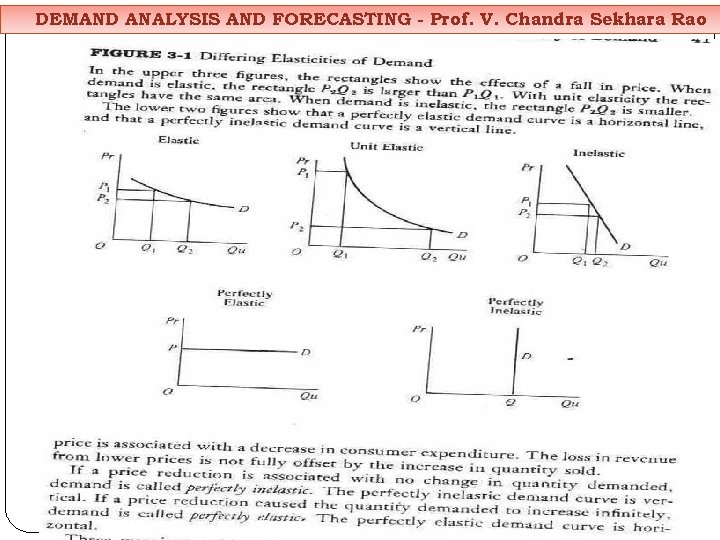DEMAND ANALYSIS AND FORECASTING - Prof. V. Chandra Sekhara Rao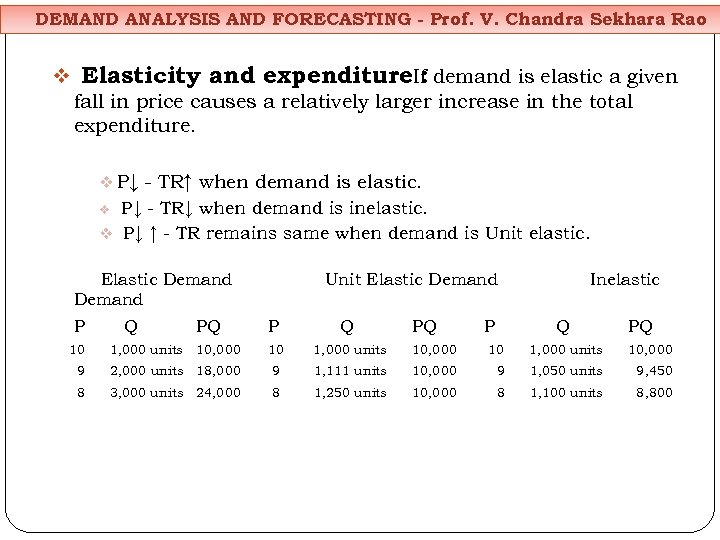DEMAND ANALYSIS AND FORECASTING - Prof. V. Chandra Sekhara Rao v Elasticity and expenditure. If demand is elastic a given : fall in price causes a relatively larger increase in the total expenditure. v P↓ - TR↑ when demand is elastic. P↓ - TR↓ when demand is inelastic. v P↓ ↑ - TR remains same when demand is Unit elastic. v Elastic Demand P Q PQ Unit Elastic Demand P Q PQ P Inelastic Q PQ 10 1, 000 units 10, 000 9 2, 000 units 18, 000 9 1, 111 units 10, 000 9 1, 050 units 9, 450 8 3, 000 units 24, 000 8 1, 250 units 10, 000 8 1, 100 units 8, 800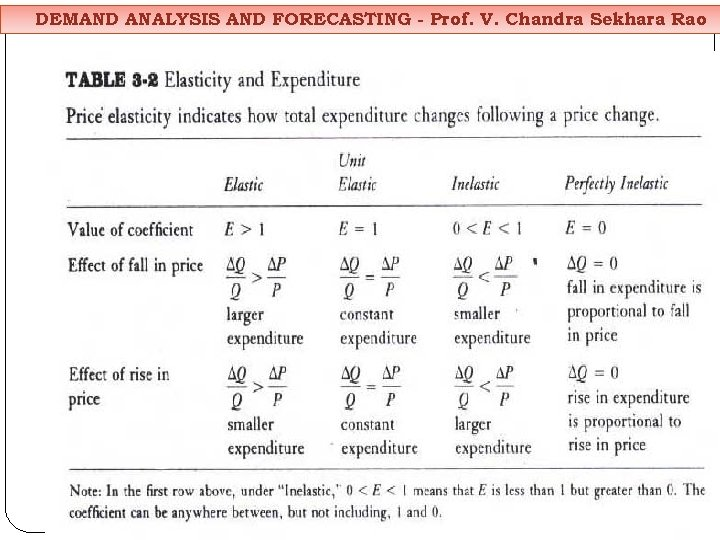DEMAND ANALYSIS AND FORECASTING - Prof. V. Chandra Sekhara Rao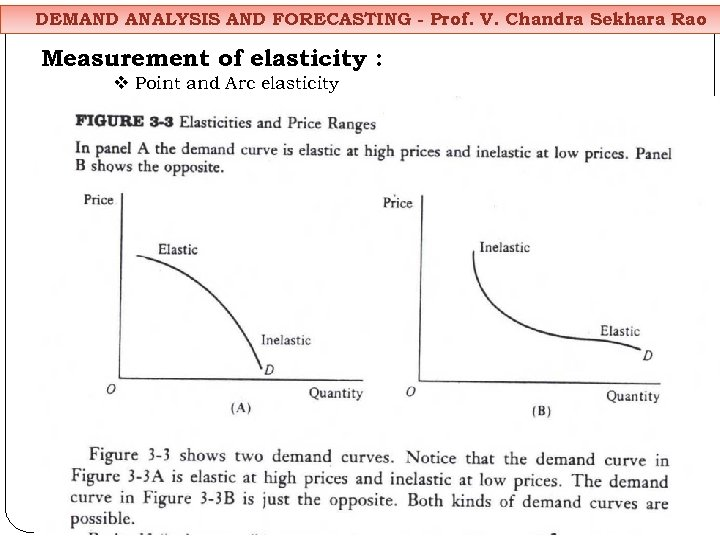DEMAND ANALYSIS AND FORECASTING - Prof. V. Chandra Sekhara Rao Measurement of elasticity : v Point and Arc elasticity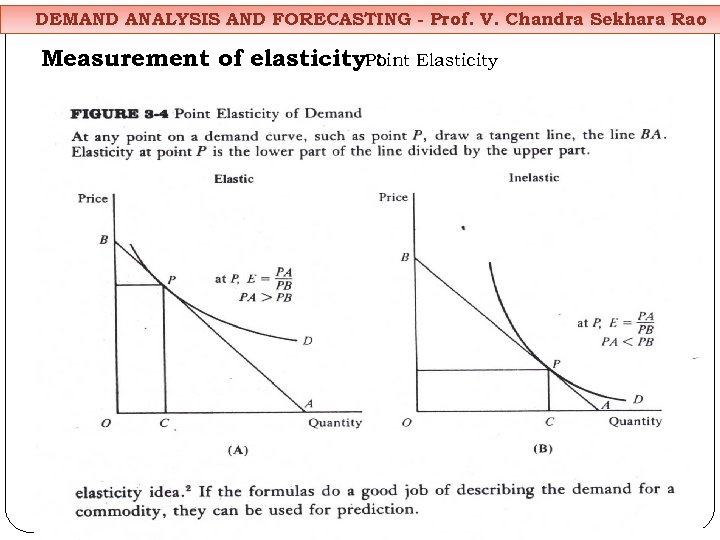DEMAND ANALYSIS AND FORECASTING - Prof. V. Chandra Sekhara Rao Measurement of elasticity. Point Elasticity :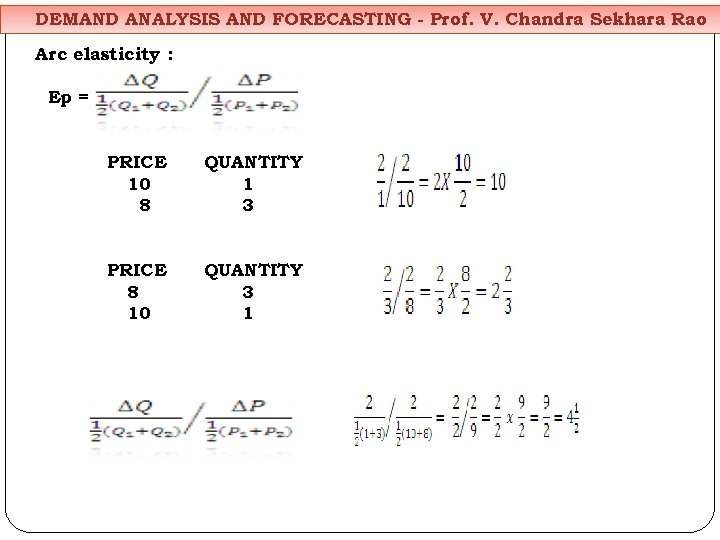DEMAND ANALYSIS AND FORECASTING - Prof. V. Chandra Sekhara Rao Arc elasticity : Ep = PRICE 10 8 QUANTITY 1 3 PRICE 8 10 QUANTITY 3 1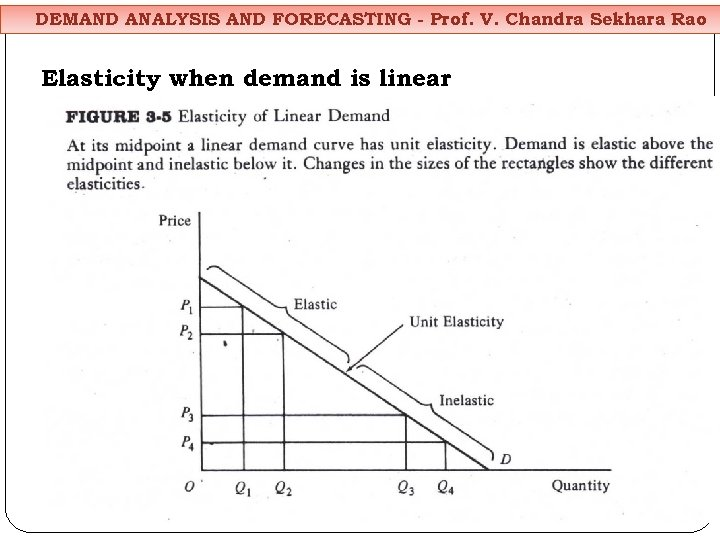DEMAND ANALYSIS AND FORECASTING - Prof. V. Chandra Sekhara Rao Elasticity when demand is linear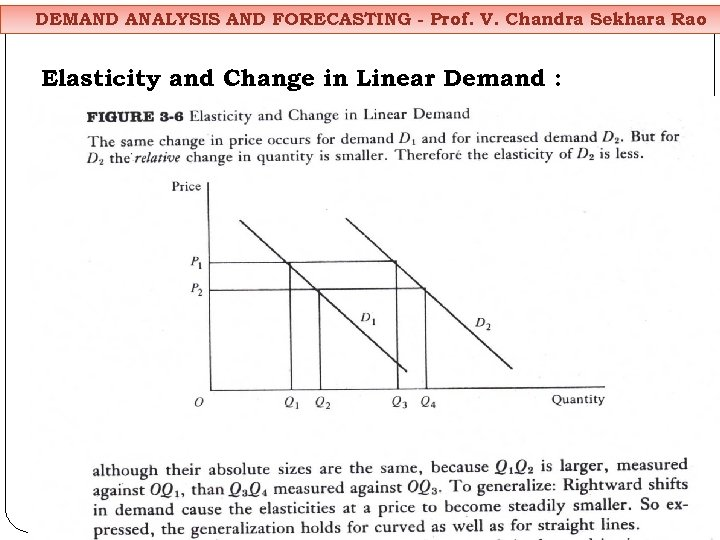DEMAND ANALYSIS AND FORECASTING - Prof. V. Chandra Sekhara Rao Elasticity and Change in Linear Demand :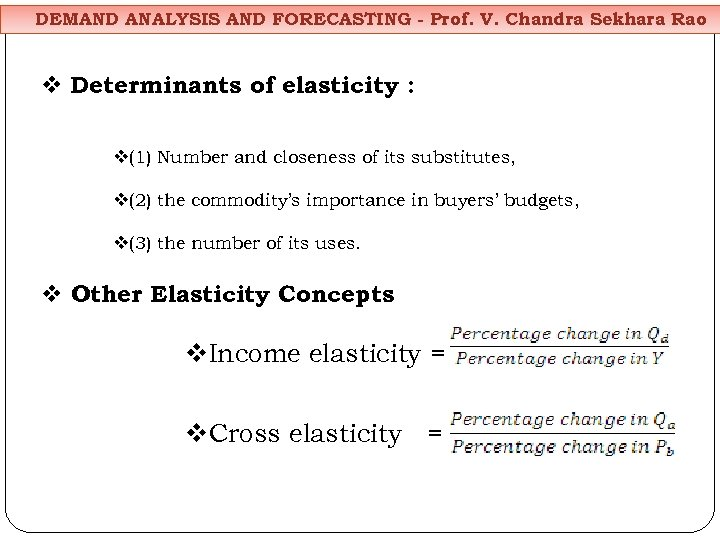DEMAND ANALYSIS AND FORECASTING - Prof. V. Chandra Sekhara Rao v Determinants of elasticity : v(1) Number and closeness of its substitutes, v(2) the commodity’s importance in buyers’ budgets, v(3) the number of its uses. v Other Elasticity Concepts v. Income elasticity = v. Cross elasticity =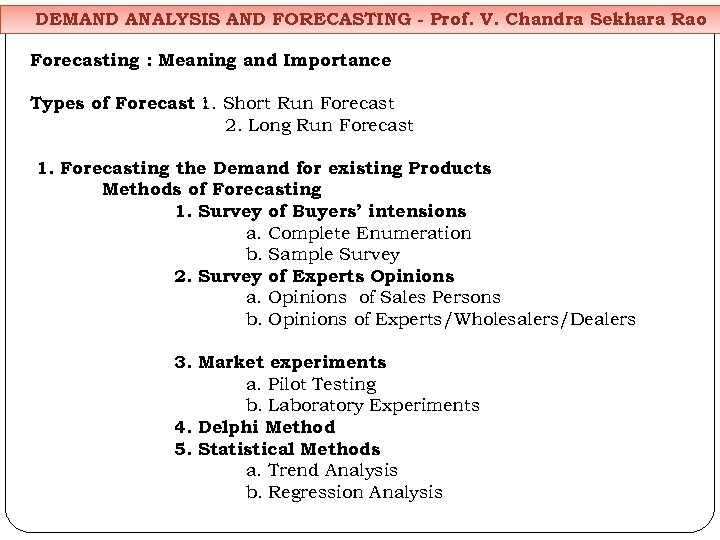DEMAND ANALYSIS AND FORECASTING - Prof. V. Chandra Sekhara Rao Forecasting : Meaning and Importance Types of Forecast 1. Short Run Forecast : 2. Long Run Forecast 1. Forecasting the Demand for existing Products Methods of Forecasting 1. Survey of Buyers’ intensions a. Complete Enumeration b. Sample Survey 2. Survey of Experts Opinions a. Opinions of Sales Persons b. Opinions of Experts/Wholesalers/Dealers 3. Market experiments a. Pilot Testing b. Laboratory Experiments 4. Delphi Method 5. Statistical Methods a. Trend Analysis b. Regression Analysis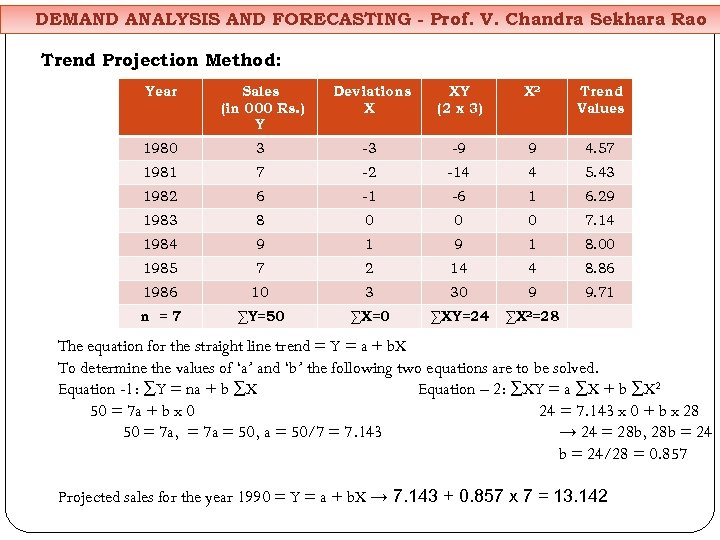DEMAND ANALYSIS AND FORECASTING - Prof. V. Chandra Sekhara Rao Trend Projection Method: Year Sales (in 000 Rs. ) Y Deviations X XY (2 x 3) X 2 Trend Values 1980 3 -3 -9 9 4. 57 1981 7 -2 -14 4 5. 43 1982 6 -1 -6 1 6. 29 1983 8 0 0 0 7. 14 1984 9 1 8. 00 1985 7 2 14 4 8. 86 1986 10 3 30 9 9. 71 n =7 ∑Y=50 ∑X=0 ∑XY=24 ∑X 2=28 The equation for the straight line trend = Y = a + b. X To determine the values of ‘a’ and ‘b’ the following two equations are to be solved. Equation -1: ∑Y = na + b ∑X Equation – 2: ∑XY = a ∑X + b ∑X 2 50 = 7 a + b x 0 24 = 7. 143 x 0 + b x 28 50 = 7 a, = 7 a = 50, a = 50/7 = 7. 143 → 24 = 28 b, 28 b = 24/28 = 0. 857 Projected sales for the year 1990 = Y = a + b. X → 7. 143 + 0. 857 x 7 = 13. 142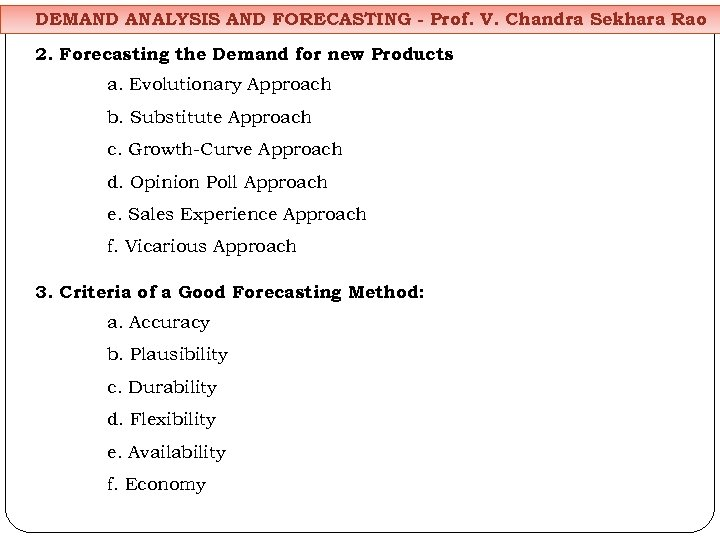DEMAND ANALYSIS AND FORECASTING - Prof. V. Chandra Sekhara Rao 2. Forecasting the Demand for new Products a. Evolutionary Approach b. Substitute Approach c. Growth-Curve Approach d. Opinion Poll Approach e. Sales Experience Approach f. Vicarious Approach 3. Criteria of a Good Forecasting Method: a. Accuracy b. Plausibility c. Durability d. Flexibility e. Availability f. Economy